SEARCH HOMEMath Central Quandaries & QueriesQuestion from Kimberly, a student: I need help with this parabola: graph y=5x^2-5x-6. I am not really understanding how to graph parabolas in general. Can you help me? Thanks, KimberlyKimberly,

I assume you know that a parabola looks like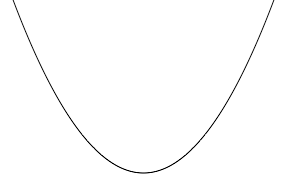or perhaps the other way up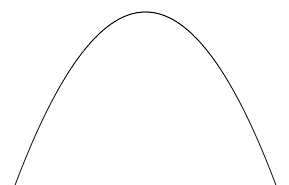or maybe sideways like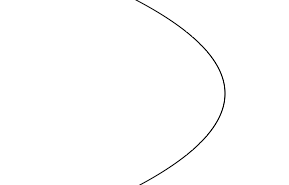or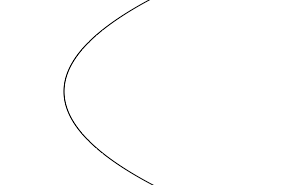or maybe even at some other angle like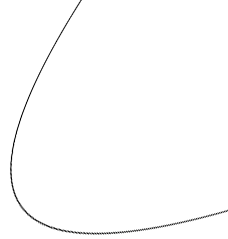or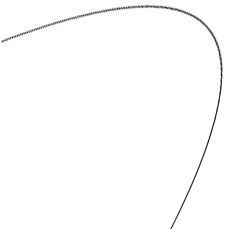I don't expect you would be asked to graph a parabola like the last two so let's stay with the first four. The challenge is to decide which of the four shapes you have and where it lies with respect to the coordinate axes.

Your parabola is y = 5x2 - 5x - 6. One point on the graph you can see easily.
If x = 0 the y = 5 × 02 - 5 × 0 - 6 = -6. Thus (0, -6) is on the graph.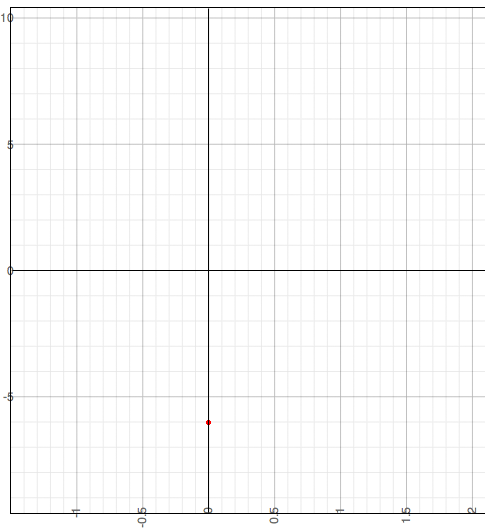You can substitute other values for x and calculate the y-values but let's try something else. What point or points on the graph have y = 0? That is for what values of x is 5x2 - 5x - 6 = 0? You can try to factor this to solve for x but it doesn't factor. Thus to solve 5x2 - 5x - 6 = 0 I need to use the general quadratic,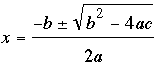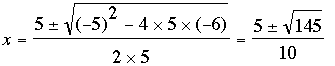Since 145 is approximately 144 and √144 = 12 I get approximate values for x as

x = -0.7 and x = 1.7.

This gives me two more points on the graph.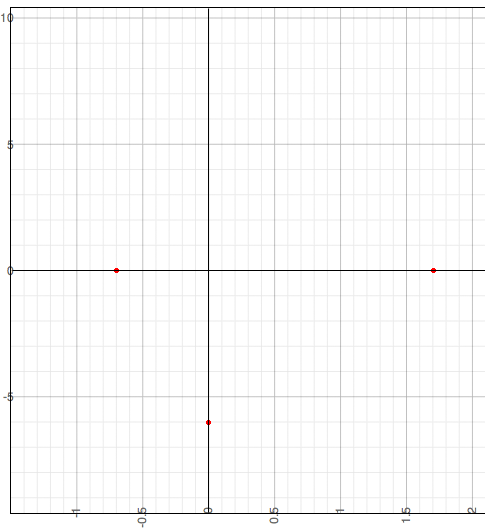Now I can see that the only option is a parabola that "opens upwards" so I have my graph.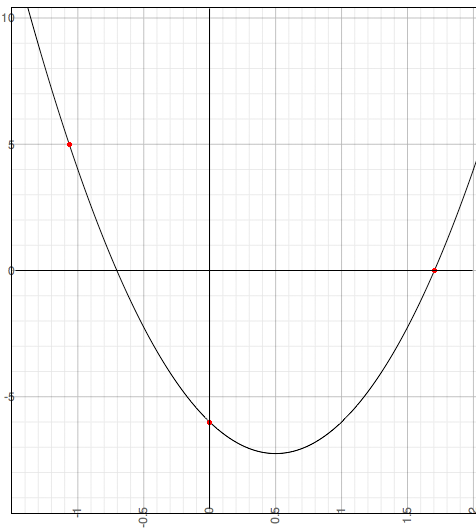I hope this helps,
PennyMath Central is supported by the University of Regina and The Pacific Institute for the Mathematical Sciences.